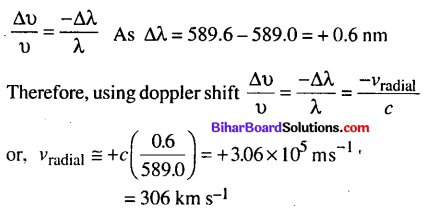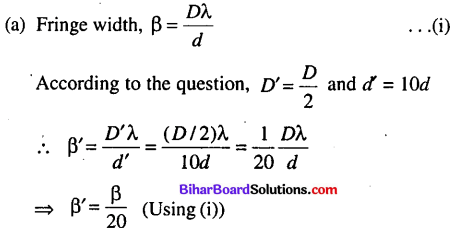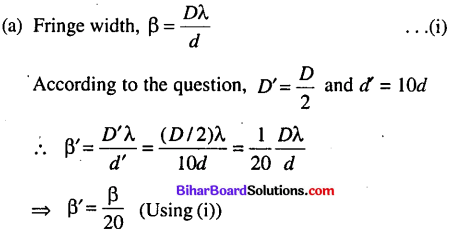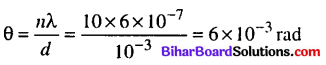# Bihar Board 12th Physics Objective Answers Chapter 10 Wave Optics

Bihar Board 12th Physics Objective Questions and Answers

## Bihar Board 12th Physics Objective Answers Chapter 10 Wave OpticsQuestion 1.
The idea of secondary wavelets for the propagation of a wave was first given by
(a) Newton
(b) Huygens
(c) Maxwell
(d) Fresnel
(b) Huygens

Question 2.
Wavefront is the locus of all points, where the particles of the medium vibrate with the same
(a) phase
(b) amplitude
(c) frequency
(d) period
(a) phase

Question 3.
Light propagates rectilinearly, due to
(a) wave nature
(b) wavelengths
(c) velocity
(d) frequency
(a) wave natureQuestion 4.
The refractive index of glass is 1,5 for light waves of λ = 6000 Å in vacuum. Its wavelength in glass is
(a) 2000 Å
(b) 4000 Å
(c) 1000 Å
(d) 3000 Å
(b) 4000 Å

Question 5.
The phenomena which is not explained by Huygen’s construction of wavefront
(a) reflection
(b) diffraction
(c) refraction
(d) origin of spectra
(d) origin of spectra

Question 6.
A plane wave passes through a convex lens. The geometrical shape of the wavefront that emerges is
(a) plane
(b) diverging spherical
(c) converging spherical
(d) none of these
(c) converging sphericalQuestion 7.
A galaxy moves with respect to us so that sodium light of 589.0 nm is observed as 589.6 nm. The speed of the galaxy is
(a) 206 km s-1
(b) 306 km s-1
(c) 103 km s-1
(d) 51km s-1
(b) 306 km s-1
Solution:
(b) The relation between υ, c and λ are, υλ = c For small changes in υ and λ ,Therefore, the galaxy is moving away from us.

Question 8.
The wavelength of spectral line coming from a distant star shifts from 600 nm to 600.1 nm. The velocity of the star relative to earth is
(a) 50 km s-1
(b) 100 km s-1
(c) 25 km s-1
(d) 200 km s-1
(a) 50 km s-1
Solution:
(a) Here, λ = 600 nm
∆λ = 600.1 nm – 600 nm = 0.1 nmQuestion 9.
A laser beam is used for locating distant objects because
(a) it is monochromatic
(b) it is constant
(c) it is not observed
(d) it has small angular spread
(d) it has small angular spreadQuestion 10.
In the case of light waves from two coherent sources S1 and S2, there will be constructive interference at an arbitrary point P, the path difference S1 P – S2P is(b) nλ

Question 11.
Which of the following is the path difference for destructive interference ?
(a) n(λ + 1)
(b) (2n + 1)$$\frac{\lambda}{2}$$
(c) nλ
(d) (n + 1)$$\frac{\lambda}{2}$$
(b) (2n + 1)$$\frac{\lambda}{2}$$

Question 12.
Two slits in Young’s double slit experiment have widths in the ratio 81:1. The ratio of the amplitudes of light waves is
(a) 3 : 1
(b) 3 : 2
(c) 9 : 1
(d) 6 : 1
(c) 9 : 1

Question 13.
The two coherent sources with intensity ratio P produce interference. The fringe visibility will be(a) $$\frac{2 \sqrt{\beta}}{1+\beta}$$

Question 14.
Young’s double slit experiment uses a monochromatic source of light. The shape of interference fringes formed on the screen is
(a) parabola
(b) straight line
(c) circle
(d) hyperbola
(d) hyperbolaQuestion 15.
When interference of light takes place
(a) energy is created in the region of maximum intensity
(b) energy is destroyed in the region of maximum intensity
(c) conservation of energy holds good and energy is redistributed
(d) conservation of energy does not hold good
(c) conservation of energy holds good and energy is redistributed

Question 16.
Two slits are made one millimeter apart and the screen is placed one metre away. The frings separation when blue green light of wavelength 500 nm is used is
(a) 5 × 10-4 m
(b) 2.5 × 10-3 m
(c) 2 × 10-4m
(d) 10 × 10-4 m
(a) 5 × 10-4 m
Solution:Question 17.
In a double slit experiment, the distance between slits is increased ten times whereas their distance from screen is halved then the fringe width is
(a) becomes $$\frac{1}{20}$$
(b) becomes $$\frac{1}{90}$$
(c) it remains same
(d) becomes $$\frac{1}{10}$$
(a) becomes $$\frac{1}{20}$$Question 18.
In a Young’s double slit experiment an electron beam is used to obtain interference pattern. If the speed of electron decreases then
(a) distance between two consecutive fringes remains the same
(b) distance between two consecutive fringes decreases
(c) distance between two consecutive fringes increases
(d) none of these
(c) distance between two consecutive fringes increases

Question 19.
In a double slit experiment using light of wavelength 600 nm, the angular width of a fringe on a distant screen is 0.1°. The spacing between the two slits is
(a) 3.44 × 10-4 m
(b) 1.54 × 10-4 m
(c) 1.54 × 10-3 m
(d) 1.44 × 10-3 m
(a) 3.44 × 10-4 m
Solution:Question 20.
In a Young’s double slit experiment, the angular width
of a fringe formed on a distant screen is 1°. The slit separation is 0.01 mm. The wavelength of the light is
(a) 0.174 nm
(b) 0.174 Å
(c) 0.174 μm
(d) 0.174 × 10-4 m
(c) 0.174 μm
Solution:
(c) Angular fringe width, θ = $$\frac{\lambda}{d}$$ ∴ λ = θd
Here, d = 0.01 mm = 10-5 m, θ = 1°= $$\frac{\pi}{180}$$ radians
λ = $$\frac{\lambda}{180}$$ × 10-5 = 1.74 × 10-7 m
= 0.174 × 10-6m = 0.174 µmQuestion 21.
In Young’s double slit experiment, the fringe width with light of wavelength 6000 A is 3 mm. The fringe width, when the wavelength of light is changed to 4000 A is
(a) 3 mm
(b) 1 mm
(c) 2 mm
(d) 4 mm
(b) 1 mm

Question 22.
The colours seen in the reflected white light from a thin oil film are due to
(a) Diffrcation
(b) Interference
(c) Polarisation
(d) Dispersion
(b) Interference

Question 23.
A slit of width a is illuminated by white light. The first minimum for red light (λ = 6500 A) will fall at θ = 30° when a will be
(a) 3200 Å
(b) 6.5 × 10-4 mm
(c) 1.3 micron
(d) 2.6 × 10-4 cm
(c) 1.3 micron

Question 24.
A parallel beam of light of wavelength 6000 A gets diffracted by a single slit of width 0.3 mm. The angular position of the first minima of diffracted light is

Question 25.
In a single slit diffraction experiment, the width of the slit is made double its original width. Then the central maximum of the diffraction pattern will become
(a) narrower and fainter
(b) narrower and brighter
(b) narrower and brighter

Question 26.
To observe diffraction, the size of the obstacle
(a) should be λ/2, where 1 is the wavelength.
(b) should be of the order of wavelength.
(c) has no relation to wavelength.
(d) should be much larger than the wavelength.
(c) has no relation to wavelength.Question 27.
A single slit is illuminated by light of wavelength 6000 A. The slit width is 0.1 cm and the screen is placed 1 m away. The angular position of 10th minimum in radian is
(a) 2 × 10-4
(b) 6 × 10-3
(c) 3 × 10-3
(d) 1 × 10-4
(b) 6 × 10-3
Solution:
(b) Here, n = 10, λ = 6000 Å = 6 × 10-7 m,
d= 0.1 cm = 10-3 m
Angular position of 10th minima.Question 28.
A diffraction pattern is obtained using a beam of red light. What happens if the red light is replaced by blue light ?
(a) No change.
(b) Diffraction bands become narrower and crowded together.
(c) Band become broader and farther apart.
(d) Bands disappear altogether.
(b) Diffraction bands become narrower and crowded together

Question 29.
In a Fresnel biprism experiment the two positions of lens give separation between the slits as 16 cm and 9 cm respectively. The actual distance of separation is
(a) 12 cm
(b) 12.5 cm
(c) 13cm
(d) 14cm
(a) 12 cm
Solution:
(a) In Fresnel biprism experiment, the actual distance of
separation between the two slits,
$$d=\sqrt{d_{1} d_{2}}=\sqrt{16 \times 9}=12 \mathrm{cm}$$

Question 30.
The angular resolution of a 10 cm diameter telescope at a wavelength of 5000 A is of the order of
Solution:Question 31.
Light of wavelength 600 nm is incident on an aperture of size 2 mm. The distance upto which light can travel such that its spread is less than the size of the aperture is
(a) 12.13 m
(b) 6.67 m
(c) 3.33 m
(d)2.19m
(b) 6.67 m

Question 32.
Transverse nature of light was confirmed by the phenomenon of
(a) refraction of light
(b) diffraction of light
(c) dispersion of light
(d) polarization of light
(d) polarization of light

Question 33.
The angle between pass axis of polarizer and analyser is 45°. The percentage of polarized light passing through analyser is
(a) 75%
(b) 25%
(c) 50%
(d) 100%
(c) 50%

Question 34.
In the case of linearly polarized light, the magnitude of the electric field vector
(a) is parallel to the direction of propagation
(b) does not change with time
(c) increases linearly with time
(d) varies periodically with time
(d) varies periodically with time

Question 35.
When ordinary light is made incident on a quarter wave plate, the emergent light is
(a) linearly polarised
(b) circularly polarised .
(c) unpolarised
(d) elliptically polarised
(d) elliptically polarisedQuestion 36.
At what angle of incidence will the light reflected from glass (μ = 1.5) be completely polarised
(a) 72.8°
(b) 51.6°
(c) 40.3°
(d) 56.3°
(d) 56.3°

Question 37.
Unpolarized light is incident on a plane glass surface. The angle of incidence so that reflected and refracted rays are perpendicular to each other, then
(a) tan iβ = μ/2
(b) tan iβ = μ
(c) sin iβ = μ
(d) cos iβ = μ
(b) tan iβ = μ

Question 38.
The refractive index of a medium is √3. If the unpolarized light is incident on it at the polarizing angle of the medium, the angle of refraction is
(a) 60°
(b) 45°
(c) 30°
(d) 0°
(c) 30°

Question 39.
The velocity of light in air is 3 x 108> m s-1 and that in water is 2.2 x 108 m s-1. The polarsing angle of incidence is
(a) 45°
(b) 50°
(c) 53.74°
(d) 63°
(c) 53.74°
Solution:
(c) The refractive index of waterFrom Brewsters law, tan ip = µ = 1.36
∴ ip = tan-1 (1.36) = 53.74°Question 40.
When the angle of incidence is 60° on the surface of a glass slab, it is found that the reflected ray is completely polarized. The velocity of light in
(a) √2 × 108ms-1
(b) √3 × 108 m s-1
(c) 2 × 108 m s-1
(d) 3 × 108 m s-1
(b) √3 × 108 m s-1
Solution:
(b) As reflected light is completely polarized, therefore, ip = 60°
ip = tan-1 = tan60°= √3Question 41.
The critical angle of a certain medium is sin-1(3/5) The polarizing angle of the medium is
(a) Sin -1(4/5)
(b) tan -1(5/3)
(c) tan -1(3/4)
(d) tan -1(4/3)
(b) tan -1(5/3)
Solution:
(b) Here, critical angle, ic = sin-1$$\left(\frac{3}{5}\right)$$
According to Brewster’s law tan ip = p where ip is the polarising angle
sinic$$\left(\frac{3}{5}\right)$$ As $$\mu=\frac{1}{\sin i_{c}}=\frac{5}{3}$$
According to brewster ‘s law tan ip = μ
where ip is the polarising angle
∴ $$\tan i_{p}=\frac{5}{3} \Rightarrow i_{p}=\tan ^{-1}\left(\frac{5}{3}\right)$$Question 42.
An optically active compound
(a) rotates the place of polarised light
(b) changes the direction of polarised light
(c) does not allow plane polarised light to pass through
(d) none of these